# Types of Trees in Data Structure

In this article, we will learn about tree and some of the common types of trees in data structure.

Tree in computer science is like a tree in the real world, the only difference is that in computer science it is visualized as upside-down with root on the top and branches originating from the root to the leaves of the tree. Tree Data Structure is used for various real-world applications as it can show relation among various nodes using the parent-child hierarchy. Due to this it is also known as hierarchical data structure. It is widely used to simplify and fasten searching and sorting operations. It is considered to be one of the most powerful and advanced data structures.

Tree is a non-linear data structure. A tree can be represented using various primitive or user defined data types. To implement tree, we can make use of arrays, linked lists, classes or other types of data structures. It is a collection of nodes that are related with each other. To show the relation, nodes are connected with edges.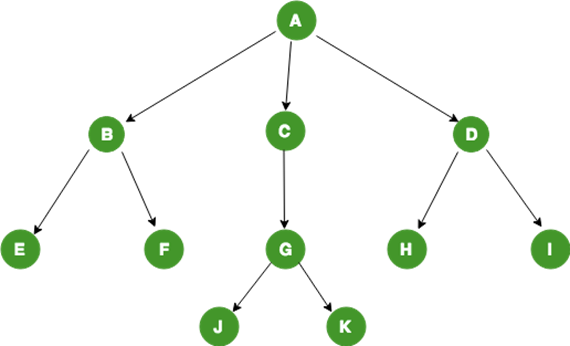Fig 1: General Tree structure (Source)

Relations in a Tree:

• A is the root of the tree
• A is Parent of B, C and D
• B is child of A
• B, C and D are siblings
• A is grand-parent of E, F, G, H and I

Properties of Tree:

• Every tree has a special node called the root node. The root node can be used to traverse every node of the tree. It is called root because the tree originated from root only.
• If a tree has N vertices(nodes) than the number of edges is always one less than the number of nodes(vertices) i.e N-1. If it has more than N-1 edges it is called a graph not a tree.
• Every child has only a single Parent but Parent can have multiple child.

## Types of Trees in Data Structure

### General Tree

A tree is called a general tree when there is no constraint imposed on the hierarchy of the tree.  In General Tree, each node can have infinite number of children. This tree is the super-set of all other types of trees. The tree shown in Fig 1 is a General Tree.

### Binary Tree

Binary tree is the type of tree in which each parent can have at most two children. The children are referred to as left child or right child. This is one of the most commonly used trees. When certain constraints and properties are imposed on Binary tree it results in a number of other widely used trees like BST (Binary Search Tree), AVL tree, RBT tree etc. We will see all these types in details as we move ahead.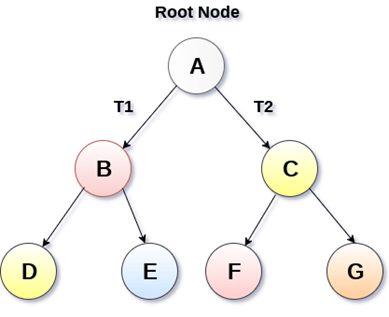Fig 2: Binary Tree (Source)

### Binary Search Tree

Binary Search Tree (BST) is an extension of Binary tree with some added constraints. In BST, the value of the left child of a node must be smaller than or equal to the value of its parent and the value of the right child is always larger than or equal to the value of its parent. This property of Binary Search Tree makes it suitable for searching operations as at each node we can decide accurately whether the value will be in left subtree or right subtree. Therefore, it is called a Search Tree.Fig 3: Binary Search Tree (Source)

### AVL Tree

AVL tree is a self-balancing binary search tree. The name AVL is given on the name of its inventors Adelson-Velshi and Landis. This was the first dynamically balancing tree. In AVL tree, each node is assigned a balancing factor based on which it is calculated whether the tree is balanced or not. In AVL tree, the heights of children of a node differ by at most 1. The valid balancing factor in AVL tree are 1, 0 and -1.  When a new node is added to the AVL tree and tree becomes unbalanced then rotation is done to make sure that the tree remains balanced. The common operations like lookup, insertion and deletion takes O(log n) time in AVL tree. It is widely used for Lookup operations.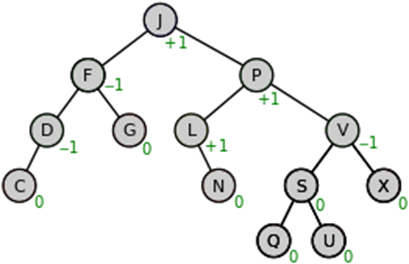Fig 4: Source

### Red-Black Tree

Red-Black is another type of self-balancing tree. The name Red-Black is given to it because each node in a Red-Black tree is either painted Red or Black according to the properties of the Red- Black Tree. This make sure that the tree remains balanced. Although the Red-Black tree is not a perfectly balanced tree but its properties ensure that the searching operation takes only O(log n) time. Whenever a new node is added to the Red-Black Tree, the nodes are rotated and painted again if needed to maintain the properties of the Red-Black Tree .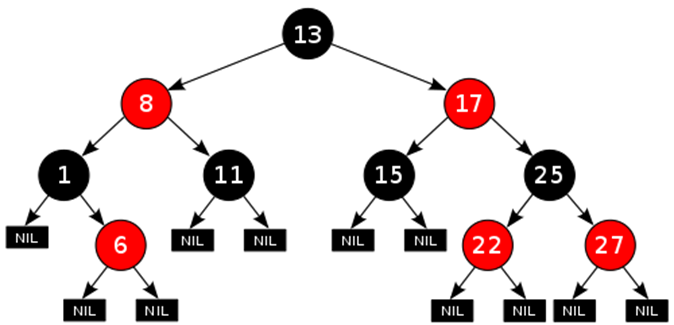Fig 5: Red-Black Tree (Source)

### N-ary Tree

In an N-ary tree, the maximum number of children that a node can have is limited to N. A binary tree is 2-ary tree as each node in binary tree has at most 2 children. Trie data structure is one of the most commonly used implementation of N-ary tree. A full N-ary tree is a tree in which children of a node is either 0 or N. A complete N-ary tree is the tree in which all the leaf nodes are at the same level.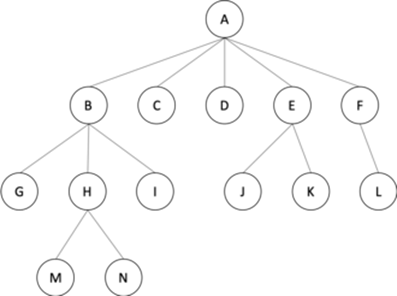Fig 6: N-ary tree (5-ary)

I hope you got the idea about some of the common types of trees in data structure. If you have any queries then feel free to ask in the comment section.